# ILP Part 19 — Students Placement Problem Part 2 — Necessary constraints

This is the nineteenth part of the ILP series. For your convenience you can find other parts in the table of contents in Part 1 – Boolean algebra

In previous part we introduced Students Placement Problem. Today we start implementing it using ILP. We will define the variables and consider constraints which are required. We need to fulfill them so the students will be able to finish their courses (so they will be physically able to attend classes and pass exams), however, we don’t care about happiness for now.

# Variables

Students Placement Problem is another example of problem easily represented using 0-1 linear programming. For every student, every course, and every class we need to answer simple question: “is student assigned to this class?” This means that we can use binary variables to represent allocations. We define the following: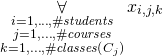In the solution, value one of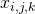means that the studentis assigned to class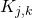. Value zero means the opposite.

Having these variables we are now able to consider constraints. We assume that every course lasts for some weeks and every week is a single unit. So student registered for such a course should attend exactly one class of that course every week. We also require that there is enough room for every assigned student. Students are allowed to mark some classes as impossible and we require they are not assigned to them. Finally, student cannot be assigned to two classes happening at the same time — we don’t want the student to learn bi-locating.

These requirements guarantees that every assigned student is physically able to finish his or her studies. The student probably will not be happy with the outcome, however, it is not our goal for now. We will take care of that later.

# Enough Classes for each Student

We require that the student is assigned to one and only one class for every course. In order to do that, we need the following constraints: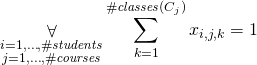For every student and for every course we sum the student’s variables for all classes of that course. We then set this sum to be equal to one which means that the student will be allocated to exactly one class within the course.

# Collisions

We do not want students to be assigned to two classes at the same time. In order to do that, for each pair of classes happening at the same moment we need to add the following constraints: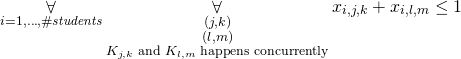You might wonder why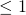instead of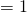. However, the latter would require the student to be assigned to at least one of the colliding classes but this is not what we want.

# Upper Bound for Assigned People

Every room has its capacity. We cannot assign more students that the room can accept because some of the students would not have a place to seat or computer to work on. So we need to add the following: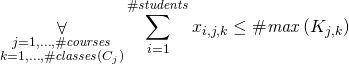In this formula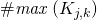means the upper bound for number of assigned students for class# Impossibility

Finally, every student is allowed to mark a class as impossible. We need to make sure that the student is not assigned to that class and we can achieve this by simply setting variable to zero: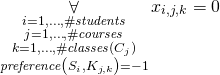# Summary

We might now try to solve the problem and we should get correct assignment for students. In next parts we will try to make sure that they get classes which they prefer. See you soon.